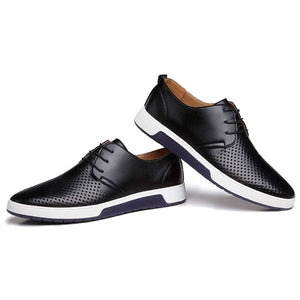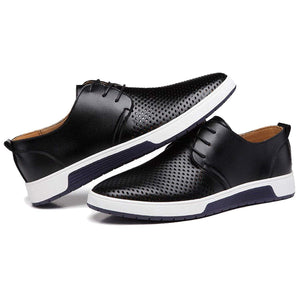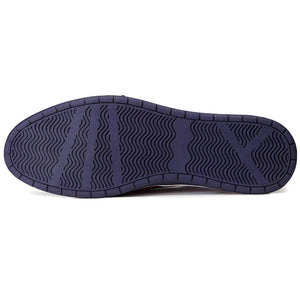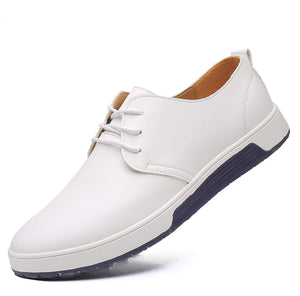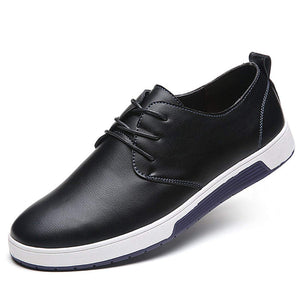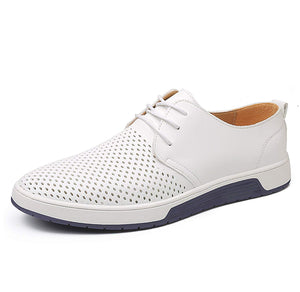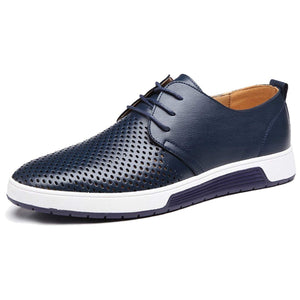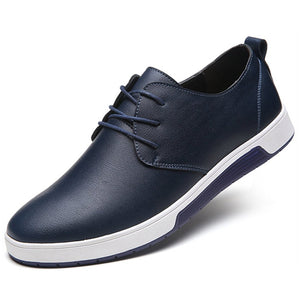# Sale

Regular price
\$40.00
Sale price
\$40.00

It's great to dress with a shirt for almost any occasion, whether formal or just casual.
Size Chart:
US size 7=CN size 40=25cm, US size 8=CN size 41=25.5cm, US size 8.5=CN size 42=26cm,
US size 9=CN size 43=26.5cm, US size 10=CN size 44=27.0cm, US size 10.5=CN size 45=27.5cm,
US size 11=CN size 46=28.0cm, US size 11.5=CN size 47=28.5cm, US size 12=CN size 48=29.0cm